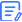1. Home
2. > Blog
3. > 43 Terms for Metal Material Mechanical Performance

# 43 Terms for Metal Material Mechanical Performance

Updated : Sep. 07, 2023

Resources

Quick Quote

## 1. Elastic limit

The maximum stress a material can withstand without experiencing permanent strain upon stress release.

## 2. Elastic modulus

The ratio of stress below the proportional limit to the corresponding strain; Young's modulus is a specific case under linear stress-strain conditions.

## 3. Poisson's ratio

The negative ratio of transverse strain generated by axial stress below the material's proportional limit to the corresponding axial strain.

## 4. Strain

The unit change in dimensions and shape of a specimen caused by external forces.

## 5. Engineering strain

The axial change in the original length relative to the original length.

## 6. Linear strain

The strain component in a given linear direction.

## 7. True strain

The natural logarithm of the instantaneous length-to-original length ratio before necking occurs.

## 8. Stress

The force or force component acting at a point on a specimen in a given plane divided by the strength at that point.

## 9. Engineering stress

Axial stress calculated based on the original cross-sectional area, the ratio of applied force to the original cross-sectional area.

## 10. Creep curve

The strain-time relationship curve during a creep test.

## 11. Creep rupture time

Time required for a specimen to deform under defined tensile stress until rupture at a specified temperature.

## 12. Creep strength

Stress causing defined strain during constant temperature and time in a creep test.

## 13. Elongation

Percentage ratio of the elongation to the original gauge length or reference length.

## 14. Creep rupture elongation

Percentage ratio of the permanent reference length increase to the original reference length after creep rupture.

## 15. Initial plastic elongation

Non-proportional increase in reference length due to applied test force, expressed as a percentage of the reference length.

## 16. Reduction of area

Percentage ratio of the maximum reduction in cross-sectional area after fracture to the original cross-sectional area.

## 17. Tensile strength

The stress corresponding to the maximum force endured before fracture of the specimen.

## 18. Compressive strength

For brittle materials, the maximum compressive stress during fracture; for ductile materials that fail without crushing during compression, compressive strength depends on prescribed strain and specimen geometry.

## 19. Shear strength

Shear stress corresponding to the maximum torsional moment.

## 20. Yield strength

Stress at which plastic deformation occurs without further increase in force in materials showing a yield phenomenon.

## 21. Upper yield strength

The highest stress value before the initial decrease in force.

## 22. Lower yield strength

The lowest stress value during yielding, excluding initial transient effects.

## 23. Compressive stress

Actual compressive force on the specimen during testing divided by its original cross-sectional area.

## 24. Compressive yield strength

Compressive stress at which plastic deformation continues without force increase during testing.

## 25. Compressive elastic modulus

Compressive stress-to-strain ratio when stress-strain relationship is linear during testing.

## 26. Endurance limit

The maximum stress a specimen can endure for a defined time without fracturing at a specified temperature.

## 27. Endurance rupture elongation

Percentage ratio of post-endurance rupture elongation to the original reference length at room temperature.

## 28. Endurance reduction of area

Percentage ratio of the maximum reduction in cross-sectional area after endurance rupture to the original cross-sectional area.

## 29. Endurance notch sensitivity coefficient

Ratio of stress at the same endurance time for notched and smooth specimens or the ratio of endurance time at the same stress.

## 30. Relaxation stress

The decrease in stress on the specimen at any given time during stress relaxation testing, i.e., the difference between initial stress and residual stress.

## 31. Relaxation stress rate

Rate of stress reduction per unit time, the slope of the stress relaxation curve at a given moment.

## 32. Brinell hardness

Material's resistance to permanent indentation deformation caused by applying test force with a hard alloy ball indenter.

## 33. Indentation hardness

Specific value expressed in units of average pressure exerted by a prescribed geometric shape and sized indenter under specific test conditions and within a specified number of test cycles.

## 34. Vickers hardness

Material's resistance to permanent indentation deformation caused by applying test force with a diamond pyramid indenter.

## 35. Rockwell hardness

Material's resistance to permanent indentation deformation caused by applying test force with a diamond pyramid or a steel ball indenter or a diamond cone indenter corresponding to a certain scale.

## 36. Shore hardness

Material's resistance to permanent indentation deformation caused by applying test force with a hard alloy or steel ball indenter, or a diamond cone indenter corresponding to a specific scale.

## 37. Brinell hardness

Material's resistance to permanent indentation deformation caused by applying test force with a diamond tetrahedral pyramid indenter.

## 38. Leeb hardness

Hardness calculated based on the ratio of the rebound velocity of a striker with a specified mass at a given velocity to the impact velocity at a distance of 1mm from the specimen's surface.

## 39. Impact absorption energy

Energy value indicated by a pointer or other indicator during an impact test, expressed as VU.

## 40. Brittle fracture percentage

Percentage of the fracture area showing brittle characteristics in relation to the total fracture area of the specimen.

## 41. Ductile fracture percentage

Percentage of the fracture area showing ductile characteristics in relation to the total fracture area of the specimen.

## 42. Shear fracture percentage

Quantitative evaluation of the fracture surface characteristics near the notch root (initiation region), remaining ligament, and final fracture region on both sides of a fractured Charpy V-notch impact specimen, used to estimate the ductility of body-centered cubic iron-based alloys.

## 43. Fracture toughness

Please note that these translations are quite technical, and some terms may have specific meanings in the context of material science and mechanics. If you have any questions about specific terms or concepts, feel free to ask!

Do you have the aluminum you need?

•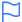•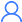•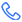•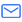•